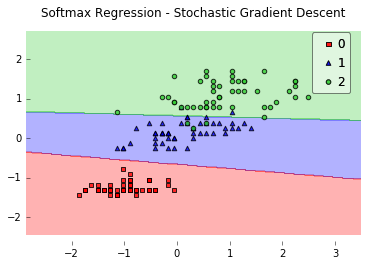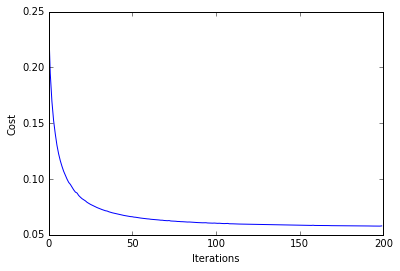Note that the optional watermark extension is a small IPython notebook plugin that I developed to make the code reproducible. You can just skip the following line(s).

In :
%load_ext watermark
%watermark -a 'Sebastian Raschka' -u -d -v -p matplotlib,numpy,scipy

Sebastian Raschka
last updated: 2016-06-05

CPython 3.5.1
IPython 4.2.0

matplotlib 1.5.1
numpy 1.11.0
scipy 0.17.0

In :
# to install watermark just uncomment the following line:
#%install_ext https://raw.githubusercontent.com/rasbt/watermark/master/watermark.py

In :
%matplotlib inline


# Bonus Material - Softmax Regression¶

Softmax Regression (synonyms: Multinomial Logistic, Maximum Entropy Classifier, or just Multi-class Logistic Regression) is a generalization of logistic regression that we can use for multi-class classification (under the assumption that the classes are mutually exclusive). In contrast, we use the (standard) Logistic Regression model in binary classification tasks.

Below is a schematic of a Logistic Regression model that we discussed in Chapter 3.In Softmax Regression (SMR), we replace the sigmoid logistic function by the so-called softmax function $\phi_{softmax}(\cdot)$.

$$P(y=j \mid z^{(i)}) = \phi_{softmax}(z^{(i)}) = \frac{e^{z^{(i)}}}{\sum_{j=0}^{k} e^{z_{k}^{(i)}}},$$

where we define the net input z as

$$z = w_1x_1 + ... + w_mx_m + b= \sum_{l=0}^{m} w_l x_l + b= \mathbf{w}^T\mathbf{x} + b.$$

(w is the weight vector, $\mathbf{x}$ is the feature vector of 1 training sample, and $b$ is the bias unit.)
Now, this softmax function computes the probability that this training sample $\mathbf{x}^{(i)}$ belongs to class $j$ given the weight and net input $z^{(i)}$. So, we compute the probability $p(y = j \mid \mathbf{x^{(i)}; w}_j)$ for each class label in $j = 1, \ldots, k.$. Note the normalization term in the denominator which causes these class probabilities to sum up to one.To illustrate the concept of softmax, let us walk through a concrete example. Let's assume we have a training set consisting of 4 samples from 3 different classes (0, 1, and 2)

• $x_0 \rightarrow \text{class }0$
• $x_1 \rightarrow \text{class }1$
• $x_2 \rightarrow \text{class }2$
• $x_3 \rightarrow \text{class }2$
In :
import numpy as np
y = np.array([0, 1, 2, 2])


First, we want to encode the class labels into a format that we can more easily work with; we apply one-hot encoding:

In :
y_enc = (np.arange(np.max(y) + 1) == y[:, None]).astype(float)
print('one-hot encoding:\n', y_enc)

one-hot encoding:
[[ 1.  0.  0.]
[ 0.  1.  0.]
[ 0.  0.  1.]
[ 0.  0.  1.]]


A sample that belongs to class 0 (the first row) has a 1 in the first cell, a sample that belongs to class 2 has a 1 in the second cell of its row, and so forth.

Next, let us define the feature matrix of our 4 training samples. Here, we assume that our dataset consists of 2 features; thus, we create a 4x2 dimensional matrix of our samples and features. Similarly, we create a 2x3 dimensional weight matrix (one row per feature and one column for each class).

In :
X = np.array([[0.1, 0.5],
[1.1, 2.3],
[-1.1, -2.3],
[-1.5, -2.5]])

W = np.array([[0.1, 0.2, 0.3],
[0.1, 0.2, 0.3]])

bias = np.array([0.01, 0.1, 0.1])

print('Inputs X:\n', X)
print('\nWeights W:\n', W)
print('\nbias:\n', bias)

Inputs X:
[[ 0.1  0.5]
[ 1.1  2.3]
[-1.1 -2.3]
[-1.5 -2.5]]

Weights W:
[[ 0.1  0.2  0.3]
[ 0.1  0.2  0.3]]

bias:
[ 0.01  0.1   0.1 ]


To compute the net input, we multiply the 4x2 matrix feature matrix X with the 2x3 (n_features x n_classes) weight matrix W, which yields a 4x3 output matrix (n_samples x n_classes) to which we then add the bias unit:

$$\mathbf{Z} = \mathbf{X}\mathbf{W} + \mathbf{b}.$$
In :
X = np.array([[0.1, 0.5],
[1.1, 2.3],
[-1.1, -2.3],
[-1.5, -2.5]])

W = np.array([[0.1, 0.2, 0.3],
[0.1, 0.2, 0.3]])

bias = np.array([0.01, 0.1, 0.1])

print('Inputs X:\n', X)
print('\nWeights W:\n', W)
print('\nbias:\n', bias)

Inputs X:
[[ 0.1  0.5]
[ 1.1  2.3]
[-1.1 -2.3]
[-1.5 -2.5]]

Weights W:
[[ 0.1  0.2  0.3]
[ 0.1  0.2  0.3]]

bias:
[ 0.01  0.1   0.1 ]

In :
def net_input(X, W, b):
return (X.dot(W) + b)

net_in = net_input(X, W, bias)
print('net input:\n', net_in)

net input:
[[ 0.07  0.22  0.28]
[ 0.35  0.78  1.12]
[-0.33 -0.58 -0.92]
[-0.39 -0.7  -1.1 ]]


Now, it's time to compute the softmax activation that we discussed earlier:

$$P(y=j \mid z^{(i)}) = \phi_{softmax}(z^{(i)}) = \frac{e^{z^{(i)}}}{\sum_{j=0}^{k} e^{z_{k}^{(i)}}}.$$
In :
def softmax(z):
return (np.exp(z.T) / np.sum(np.exp(z), axis=1)).T

smax = softmax(net_in)
print('softmax:\n', smax)

softmax:
[[ 0.29450637  0.34216758  0.36332605]
[ 0.21290077  0.32728332  0.45981591]
[ 0.42860913  0.33380113  0.23758974]
[ 0.44941979  0.32962558  0.22095463]]


As we can see, the values for each sample (row) nicely sum up to 1 now. E.g., we can say that the first sample
[ 0.29450637 0.34216758 0.36332605] has a 29.45% probability to belong to class 0.

Now, in order to turn these probabilities back into class labels, we could simply take the argmax-index position of each row:

[[ 0.29450637 0.34216758 0.36332605] -> 2
[ 0.21290077 0.32728332 0.45981591] -> 2
[ 0.42860913 0.33380113 0.23758974] -> 0
[ 0.44941979 0.32962558 0.22095463]] -> 0

In :
def to_classlabel(z):
return z.argmax(axis=1)

print('predicted class labels: ', to_classlabel(smax))

predicted class labels:  [2 2 0 0]


As we can see, our predictions are terribly wrong, since the correct class labels are [0, 1, 2, 2]. Now, in order to train our logistic model (e.g., via an optimization algorithm such as gradient descent), we need to define a cost function $J(\cdot)$ that we want to minimize:

$$J(\mathbf{W}; \mathbf{b}) = \frac{1}{n} \sum_{i=1}^{n} H(T_i, O_i),$$

which is the average of all cross-entropies over our $n$ training samples. The cross-entropy function is defined as

$$H(T_i, O_i) = -\sum_m T_i \cdot log(O_i).$$

Here the $T$ stands for "target" (i.e., the true class labels) and the $O$ stands for output -- the computed probability via softmax; not the predicted class label.

In :
def cross_entropy(output, y_target):
return - np.sum(np.log(output) * (y_target), axis=1)

xent = cross_entropy(smax, y_enc)
print('Cross Entropy:', xent)

Cross Entropy: [ 1.22245465  1.11692907  1.43720989  1.50979788]

In :
def cost(output, y_target):
return np.mean(cross_entropy(output, y_target))

J_cost = cost(smax, y_enc)
print('Cost: ', J_cost)

Cost:  1.32159787159


In order to learn our softmax model -- determining the weight coefficients -- via gradient descent, we then need to compute the derivative

$$\nabla \mathbf{w}_j \, J(\mathbf{W}; \mathbf{b}).$$

I don't want to walk through the tedious details here, but this cost derivative turns out to be simply:

$$\nabla \mathbf{w}_j \, J(\mathbf{W}; \mathbf{b}) = \frac{1}{n} \sum^{n}_{i=0} \big[\mathbf{x}^{(i)}\ \big(O_i - T_i \big) \big]$$

We can then use the cost derivate to update the weights in opposite direction of the cost gradient with learning rate $\eta$:

$$\mathbf{w}_j := \mathbf{w}_j - \eta \nabla \mathbf{w}_j \, J(\mathbf{W}; \mathbf{b})$$

for each class $$j \in \{0, 1, ..., k\}$$

(note that $\mathbf{w}_j$ is the weight vector for the class $y=j$), and we update the bias units

$$\mathbf{b}_j := \mathbf{b}_j - \eta \bigg[ \frac{1}{n} \sum^{n}_{i=0} \big(O_i - T_i \big) \bigg].$$

As a penalty against complexity, an approach to reduce the variance of our model and decrease the degree of overfitting by adding additional bias, we can further add a regularization term such as the L2 term with the regularization parameter $\lambda$:

L2: $\frac{\lambda}{2} ||\mathbf{w}||_{2}^{2}$,

where

$$||\mathbf{w}||_{2}^{2} = \sum^{m}_{l=0} \sum^{k}_{j=0} w_{i, j}$$

so that our cost function becomes

$$J(\mathbf{W}; \mathbf{b}) = \frac{1}{n} \sum_{i=1}^{n} H(T_i, O_i) + \frac{\lambda}{2} ||\mathbf{w}||_{2}^{2}$$

and we define the "regularized" weight update as

$$\mathbf{w}_j := \mathbf{w}_j - \eta \big[\nabla \mathbf{w}_j \, J(\mathbf{W}) + \lambda \mathbf{w}_j \big].$$

(Please note that we don't regularize the bias term.)

# SoftmaxRegression Code¶

Bringing the concepts together, we could come up with an implementation as follows:

In :
# Sebastian Raschka 2016
# Implementation of the mulitnomial logistic regression algorithm for
# classification.

# Author: Sebastian Raschka <sebastianraschka.com>
#

import numpy as np
from time import time
#from .._base import _BaseClassifier
#from .._base import _BaseMultiClass

class SoftmaxRegression(object):

"""Softmax regression classifier.

Parameters
------------
eta : float (default: 0.01)
Learning rate (between 0.0 and 1.0)
epochs : int (default: 50)
Passes over the training dataset.
Prior to each epoch, the dataset is shuffled
if minibatches > 1 to prevent cycles in stochastic gradient descent.
l2 : float
Regularization parameter for L2 regularization.
No regularization if l2=0.0.
minibatches : int (default: 1)
The number of minibatches for gradient-based optimization.
If len(y): Stochastic Gradient Descent (SGD) online learning
If 1 < minibatches < len(y): SGD Minibatch learning
n_classes : int (default: None)
A positive integer to declare the number of class labels
if not all class labels are present in a partial training set.
Gets the number of class labels automatically if None.
random_seed : int (default: None)
Set random state for shuffling and initializing the weights.

Attributes
-----------
w_ : 2d-array, shape={n_features, 1}
Model weights after fitting.
b_ : 1d-array, shape={1,}
Bias unit after fitting.
cost_ : list
List of floats, the average cross_entropy for each epoch.

"""
def __init__(self, eta=0.01, epochs=50,
l2=0.0,
minibatches=1,
n_classes=None,
random_seed=None):

self.eta = eta
self.epochs = epochs
self.l2 = l2
self.minibatches = minibatches
self.n_classes = n_classes
self.random_seed = random_seed

def _fit(self, X, y, init_params=True):
if init_params:
if self.n_classes is None:
self.n_classes = np.max(y) + 1
self._n_features = X.shape

self.b_, self.w_ = self._init_params(
weights_shape=(self._n_features, self.n_classes),
bias_shape=(self.n_classes,),
random_seed=self.random_seed)
self.cost_ = []

y_enc = self._one_hot(y=y, n_labels=self.n_classes, dtype=np.float)

for i in range(self.epochs):
for idx in self._yield_minibatches_idx(
n_batches=self.minibatches,
data_ary=y,
shuffle=True):
# givens:
# w_ -> n_feat x n_classes
# b_  -> n_classes

# net_input, softmax and diff -> n_samples x n_classes:
net = self._net_input(X[idx], self.w_, self.b_)
softm = self._softmax(net)
diff = softm - y_enc[idx]
mse = np.mean(diff, axis=0)

# gradient -> n_features x n_classes

# update in opp. direction of the cost gradient
self.w_ -= (self.eta * grad +
self.eta * self.l2 * self.w_)
self.b_ -= (self.eta * np.sum(diff, axis=0))

# compute cost of the whole epoch
net = self._net_input(X, self.w_, self.b_)
softm = self._softmax(net)
cross_ent = self._cross_entropy(output=softm, y_target=y_enc)
cost = self._cost(cross_ent)
self.cost_.append(cost)
return self

def fit(self, X, y, init_params=True):
"""Learn model from training data.

Parameters
----------
X : {array-like, sparse matrix}, shape = [n_samples, n_features]
Training vectors, where n_samples is the number of samples and
n_features is the number of features.
y : array-like, shape = [n_samples]
Target values.
init_params : bool (default: True)
Re-initializes model parametersprior to fitting.
Set False to continue training with weights from
a previous model fitting.

Returns
-------
self : object

"""
if self.random_seed is not None:
np.random.seed(self.random_seed)
self._fit(X=X, y=y, init_params=init_params)
self._is_fitted = True
return self

def _predict(self, X):
probas = self.predict_proba(X)
return self._to_classlabels(probas)

def predict(self, X):
"""Predict targets from X.

Parameters
----------
X : {array-like, sparse matrix}, shape = [n_samples, n_features]
Training vectors, where n_samples is the number of samples and
n_features is the number of features.

Returns
----------
target_values : array-like, shape = [n_samples]
Predicted target values.

"""
if not self._is_fitted:
raise AttributeError('Model is not fitted, yet.')
return self._predict(X)

def predict_proba(self, X):
"""Predict class probabilities of X from the net input.

Parameters
----------
X : {array-like, sparse matrix}, shape = [n_samples, n_features]
Training vectors, where n_samples is the number of samples and
n_features is the number of features.

Returns
----------
Class probabilties : array-like, shape= [n_samples, n_classes]

"""
net = self._net_input(X, self.w_, self.b_)
softm = self._softmax(net)
return softm

def _net_input(self, X, W, b):
return (X.dot(W) + b)

def _softmax(self, z):
return (np.exp(z.T) / np.sum(np.exp(z), axis=1)).T

def _cross_entropy(self, output, y_target):
return - np.sum(np.log(output) * (y_target), axis=1)

def _cost(self, cross_entropy):
L2_term = self.l2 * np.sum(self.w_ ** 2)
cross_entropy = cross_entropy + L2_term
return 0.5 * np.mean(cross_entropy)

def _to_classlabels(self, z):
return z.argmax(axis=1)

def _init_params(self, weights_shape, bias_shape=(1,), dtype='float64',
scale=0.01, random_seed=None):
"""Initialize weight coefficients."""
if random_seed:
np.random.seed(random_seed)
w = np.random.normal(loc=0.0, scale=scale, size=weights_shape)
b = np.zeros(shape=bias_shape)
return b.astype(dtype), w.astype(dtype)

def _one_hot(self, y, n_labels, dtype):
"""Returns a matrix where each sample in y is represented
as a row, and each column represents the class label in
the one-hot encoding scheme.

Example:

y = np.array([0, 1, 2, 3, 4, 2])
mc = _BaseMultiClass()
mc._one_hot(y=y, n_labels=5, dtype='float')

np.array([[1., 0., 0., 0., 0.],
[0., 1., 0., 0., 0.],
[0., 0., 1., 0., 0.],
[0., 0., 0., 1., 0.],
[0., 0., 0., 0., 1.],
[0., 0., 1., 0., 0.]])

"""
mat = np.zeros((len(y), n_labels))
for i, val in enumerate(y):
mat[i, val] = 1
return mat.astype(dtype)

def _yield_minibatches_idx(self, n_batches, data_ary, shuffle=True):
indices = np.arange(data_ary.shape)

if shuffle:
indices = np.random.permutation(indices)
if n_batches > 1:
remainder = data_ary.shape % n_batches

if remainder:
minis = np.array_split(indices[:-remainder], n_batches)
minis[-1] = np.concatenate((minis[-1],
indices[-remainder:]),
axis=0)
else:
minis = np.array_split(indices, n_batches)

else:
minis = (indices,)

for idx_batch in minis:
yield idx_batch

def _shuffle_arrays(self, arrays):
"""Shuffle arrays in unison."""
r = np.random.permutation(len(arrays))
return [ary[r] for ary in arrays]


## Example 1 - Gradient Descent¶

In :
from mlxtend.data import iris_data
from mlxtend.plotting import plot_decision_regions
import matplotlib.pyplot as plt

X, y = iris_data()
X = X[:, [0, 3]] # sepal length and petal width

# standardize
X[:,0] = (X[:,0] - X[:,0].mean()) / X[:,0].std()
X[:,1] = (X[:,1] - X[:,1].mean()) / X[:,1].std()

lr = SoftmaxRegression(eta=0.01, epochs=10, minibatches=1, random_seed=0)
lr.fit(X, y)

plot_decision_regions(X, y, clf=lr)
plt.show()

plt.plot(range(len(lr.cost_)), lr.cost_)
plt.xlabel('Iterations')
plt.ylabel('Cost')
plt.show()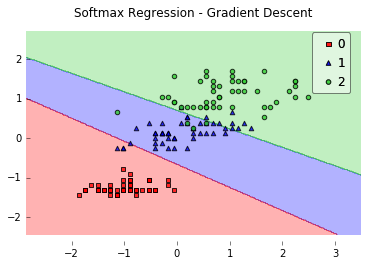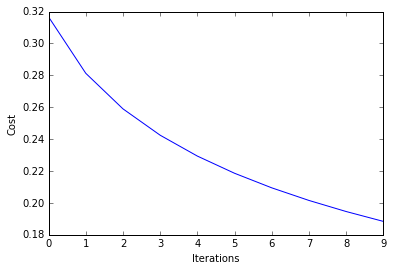Continue training for another 800 epochs by calling the fit method with init_params=False.

In :
lr.epochs = 800

lr.fit(X, y, init_params=False)

plot_decision_regions(X, y, clf=lr)
plt.title('Softmax Regression - Stochastic Gradient Descent')
plt.show()

plt.plot(range(len(lr.cost_)), lr.cost_)
plt.xlabel('Iterations')
plt.ylabel('Cost')
plt.show()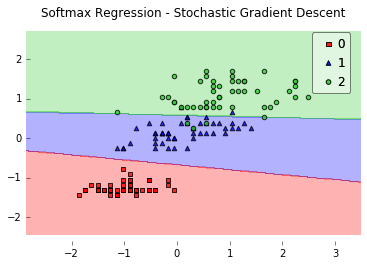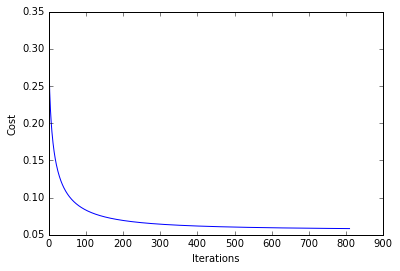### Predicting Class Labels¶

In :
y_pred = lr.predict(X)
print('Last 3 Class Labels: %s' % y_pred[-3:])

Last 3 Class Labels: [2 2 2]


### Predicting Class Probabilities¶

In :
y_pred = lr.predict_proba(X)
print('Last 3 Class Labels:\n %s' % y_pred[-3:])

Last 3 Class Labels:
[[  1.22579350e-09   1.22074818e-02   9.87792517e-01]
[  1.84962350e-12   3.99742930e-04   9.99600257e-01]
[  3.10919973e-07   1.37047172e-01   8.62952517e-01]]


## Example 2 - Stochastic Gradient Descent¶

In :
from mlxtend.data import iris_data
from mlxtend.plotting import plot_decision_regions
from mlxtend.classifier import SoftmaxRegression
import matplotlib.pyplot as plt

X, y = iris_data()
X = X[:, [0, 3]] # sepal length and petal width

# standardize
X[:,0] = (X[:,0] - X[:,0].mean()) / X[:,0].std()
X[:,1] = (X[:,1] - X[:,1].mean()) / X[:,1].std()

lr = SoftmaxRegression(eta=0.05, epochs=200, minibatches=len(y), random_seed=0)
lr.fit(X, y)

plot_decision_regions(X, y, clf=lr)
plt.title('Softmax Regression - Stochastic Gradient Descent')
plt.show()

plt.plot(range(len(lr.cost_)), lr.cost_)
plt.xlabel('Iterations')
plt.ylabel('Cost')
plt.show()